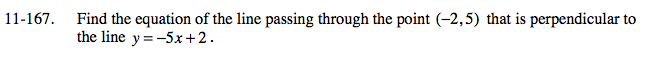### Home > A2C > Chapter 11 > Lesson 11.3.4 > Problem11-167

11-167.A perpendicular line has a slope that is the opposite reciprocal of the given line.

Using y = mx + b, substitute the m, y, and x and solve for b.

$y= \frac{ 1 }{ 5} x+ \frac{ 27 }{ 5}$